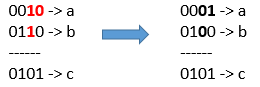# 或运算的最小翻转次数

## 1318. 或运算的最小翻转次数 (Medium)

「位翻转操作」是指将一个数的二进制表示任何单个位上的 1 变成 0 或者 0 变成 1 。```输入：a = 2, b = 6, c = 5

```输入：a = 4, b = 2, c = 7

```

```输入：a = 1, b = 2, c = 3

```

• `1 <= a <= 10^9`
• `1 <= b <= 10^9`
• `1 <= c <= 10^9`

[位运算]